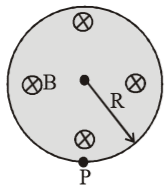• Subject:

...

• Topic:

...

A uniform magnetic field of induction $\mathrm{B}$ is confined to a cylindrical region of radius $\mathrm{R}$. The magnetic field is increasing at a constant rate of $\frac{\mathrm{dB}}{\mathrm{dt}}$ (tesla/second). An electron of charge $\mathrm{q}$, placed at the point $\mathrm{P}$ on the periphery of the field experiences an acceleration.1. $\frac{\mathrm{BV}}{\left(\sqrt{2}+1\right)\mathrm{r}}$ towards left

2. $\frac{1}{2}\frac{\mathrm{eR}}{\mathrm{m}}\frac{\mathrm{dB}}{\mathrm{dt}}$ towards right

3. $\frac{\mathrm{eR}}{\mathrm{m}}\frac{\mathrm{dB}}{\mathrm{dt}}$ towards left

4. zero